# Tableau Functions (by Category)

The Tableau functions in this reference are organized by category. Click a category to browse its functions. Or press Ctrl+F (Command-F on a Mac) to open a search box that you can use to search the page for a specific function.

Number functions

### ABS

 Syntax `ABS(number)` Output Number (positive) Definition Returns the absolute value of the given number. Example `ABS(-7) = 7ABS([Budget Variance])` The second example returns the absolute value for all the numbers contained in the Budget Variance field. Notes See also `SIGN`.

### ACOS

 Syntax `ACOS(number)` Output Number (angle in radians) Definition Returns the arccosine (angle) of the given number. Example `ACOS(-1) = 3.14159265358979` Notes The inverse function, `COS`, takes the angle in radians as the argument and returns the cosine.

### ASIN

 Syntax `ASIN(number)` Output Number (angle in radians) Definition Returns the arcsine (angle) of a given number. Example `ASIN(1) = 1.5707963267949` Notes The inverse function, `SIN`, takes the angle in radians as the argument and returns the sine.

### ATAN

 Syntax `ATAN(number)` Output Number (angle in radians) Definition Returns the arctangent (angle) of a given number. Example `ATAN(180) = 1.5652408283942` Notes The inverse function, `TAN`, takes the angle in radians as the argument and returns the tangent. See also `ATAN2` and `COT`.

### ATAN2

 Syntax `ATAN2(y number, x number)` Output Number (angle in radians) Definition Returns the arctangent (angle) between two numbers (x and y). The result is in radians. Example `ATAN2(2, 1) = 1.10714871779409` Notes See also `ATAN`, `TAN`, and `COT`.

### CEILING

 Syntax `CEILING(number)` Output Integer Definition Rounds a number to the nearest integer of equal or greater value. Example `CEILING(2.1) = 3` Notes See also `FLOOR` and `ROUND`. Database limitations `CEILING` is available through the following connectors: Microsoft Excel, Text File, Statistical File, Published Data Source, Amazon EMR Hadoop Hive, Amazon Redshift, Cloudera Hadoop, DataStax Enterprise, Google Analytics, Google BigQuery, Hortonworks Hadoop Hive, MapR Hadoop Hive, Microsoft SQL Server, Salesforce, Spark SQL.

### COS

 Syntax `COS(number)` The number argument is the angle in radians. Output Number Definition Returns the cosine of an angle. Example `COS(PI( ) /4) = 0.707106781186548` Notes The inverse function, `ACOS`, takes the cosine as the argument and returns the angle in radians. See also `PI`.

### COT

 Syntax `COT(number)` The number argument is the angle in radians. Output Number Definition Returns the cotangent of an angle. Example `COT(PI( ) /4) = 1` Notes See also `ATAN`, `TAN`, and `PI`.

### DEGREES

 Syntax `DEGREES(number)` The number argument is the angle in radians. Output Number (degrees) Definition Converts an angle in radians to degrees. Example `DEGREES(PI( )/4) = 45.0` Notes The inverse function, `RADIANS`, takes an angle in degrees and returns the angle in radians. See also `PI()`.

### DIV

 Syntax `DIV(integer1, integer2)` Output Integer Definition Returns the integer part of a division operation, in which integer1 is divided by integer2. Example `DIV(11,2) = 5`

### EXP

 Syntax `EXP(number)` Output Number Definition Returns e raised to the power of the given number. Example `EXP(2) = 7.389EXP(-[Growth Rate]*[Time])` Notes See also `LN`.

### FLOOR

 Syntax `FLOOR(number)` Output Integer Definition Rounds a number to the nearest integer of equal or lesser value. Example `FLOOR(7.9) = 7` Notes See also `CEILING` and `ROUND`. Database limitations `FLOOR` is available through the following connectors: Microsoft Excel, Text File, Statistical File, Published Data Source, Amazon EMR Hadoop Hive, Cloudera Hadoop, DataStax Enterprise, Google Analytics, Google BigQuery, Hortonworks Hadoop Hive, MapR Hadoop Hive, Microsoft SQL Server, Salesforce, Spark SQL.

### HEXBINX

 Syntax `HEXBINX(number, number)` Output Number Definition Maps an x, y coordinate to the x-coordinate of the nearest hexagonal bin. The bins have side length 1, so the inputs may need to be scaled appropriately. Example `HEXBINX([Longitude]*2.5, [Latitude]*2.5)` Notes `HEXBINX `and `HEXBINY` are binning and plotting functions for hexagonal bins. Hexagonal bins are an efficient and elegant option for visualizing data in an x/y plane such as a map. Because the bins are hexagonal, each bin closely approximates a circle and minimizes variation in the distance from the data point to the center of the bin. This makes the clustering both more accurate and informative.

### HEXBINY

 Syntax `HEXBINY(number, number)` Output Number Definition Maps an x, y coordinate to the y-coordinate of the nearest hexagonal bin. The bins have side length 1, so the inputs may need to be scaled appropriately. Example `HEXBINY([Longitude]*2.5, [Latitude]*2.5)` Notes See also `HEXBINX`.

### LN

 Syntax `LN(number)` Output Number The output is `Null` if the argument is less than or equal to zero. Definition Returns the natural logarithm of a number. Example `LN(50) = 3.912023005` Notes See also `EXP` and `LOG`.

### LOG

 Syntax `LOG(number, [base])` If the optional base argument isn't present, base 10 is used. Output Number Definition Returns the logarithm of a number for the given base. Example `LOG(16,4) = 2` Notes See also `POWER` `LN`.

### MAX

 Syntax `MAX(expression)` or `MAX(expr1, expr2)` Output Same data type as the argument, or `NULL `if any part of the argument is null. Definition Returns the maximum of the two arguments, which must be of the same data type. `MAX` can also be applied to a single field as an aggregation. Example `MAX(4,7) = 7MAX(#3/25/1986#, #2/20/2021#) = #2/20/2021# MAX([Name])` Notes For strings, the `MAX` is the value that comes last in alphabetical order. For dates, the `MAX` is the most recent date. See also `MIN`.

### MIN

 Syntax `MIN(expression)` or `MIN(expr1, expr2)` Output Same data type as the argument, or `NULL `if any part of the argument is null. Definition Returns the maximum of the two arguments, which must be of the same data type. `MIN `can also be applied to a single field as an aggregation. Example `MIN(4,7) = 7MIN(#3/25/1986#, #2/20/2021#) = #3/25/1986#MIN([Name])` Notes For strings, the `MIN` is the value that comes first in alphabetical order. For dates, the `MIN` is the earliest date. See also `MAX`.

### PI

 Syntax `PI()` Output Number Definition Returns the numeric constant pi: 3.14159... Example `PI() = 3.14159` Notes Useful for trig functions that take their input in radians.

### POWER

 Syntax `POWER(number, power)` Output Number Definition Raises the number to the specified power. Example `POWER(5,3) = 125POWER([Temperature], 2)` Notes You can also use the ^ symbol, such as `5^3 = POWER(5,3) = 125`See also `EXP`, `LOG`, and `SQUARE`.

 Syntax `RADIANS(number)` Output Number (angle in radians) Definition Converts the given number from degrees to radians. Example `RADIANS(180) = 3.14159` Notes The inverse function, `DEGREES`, takes an angle in radians and returns the angle in degrees.

### ROUND

 Syntax `ROUND(number, [decimals])` Output Number Definition Rounds numbers to a specified number of digits. The optional `decimals` argument specifies how many decimal points of precision to include in the final result. If `decimals` is omitted, number is rounded to the nearest integer. Example `ROUND(1/3, 2) = 0.33` Notes Some databases, such as SQL Server, allow specification of a negative length, where -1 rounds number to 10's, -2 rounds to 100's, and so on. This is not true of all databases. For example, it is not true of Excel or Access. Tip: Because ROUND may run into issues due to the underlying floating point representation of numbers—such as 9.405 rounding to 9.40—it may be preferable to format the number to the desired number of decimal points rather than rounding. Formatting 9.405 to two decimal places will yield the expected 9.41. See also `CEILING` and `FLOOR`.

### SIGN

 Syntax `SIGN(number)` Output -1, 0, or 1 Definition Returns the sign of a number: The possible return values are -1 if the number is negative, 0 if the number is zero, or 1 if the number is positive. Example `SIGN(AVG(Profit)) = -1` Notes See also `ABS`.

### SIN

 Syntax `SIN(number)` The number argument is the angle in radians. Output Number Definition Returns the sine of an angle. Example `SIN(0) = 1.0SIN(PI( )/4) = 0.707106781186548` Notes The inverse function, `ASIN`, takes the sine as the argument and returns the angle in radians. See also `PI`.

### SQRT

 Syntax `SQRT(number)` Output Number Definition Returns the square root of a number. Example `SQRT(25) = 5` Notes See also `SQUARE`.

### SQUARE

 Syntax `SQUARE(number)` Output Number Definition Returns the square of a number. Example `SQUARE(5) = 25` Notes See also `SQRT` and `POWER`.

### TAN

 Syntax `TAN(number)` The number argument is the angle in radians. Output Number Definition Returns the tangent of an angle. Example `TAN(PI ( )/4) = 1.0` Notes See also `ATAN`, `ATAN2`,`COT`, and `PI`.

### ZN

 Syntax `ZN(expression)` Output Any, or o Definition Returns the expression if it is not null, otherwise returns zero. Use this function to replace null values with zeros. Example `ZN(Grade) = 0` Notes This is a very useful function when using fields that may contain nulls in a calculation. Wrapping the field with `ZN` can prevent errors caused by calculating with nulls.
String functions

#### Definition

ASCII

`ASCII(string)`

Returns the ASCII code for the first character of `string`.

Example:

```ASCII('A') = 65```

CHAR

`CHAR(number)`

Returns the character encoded by the ASCII code `number`.

Example:

```CHAR(65) = 'A'```

CONTAINS

`CONTAINS(string, substring)`

Returns true if the given string contains the specified substring.

Example:

```CONTAINS(“Calculation”, “alcu”) = true```

ENDSWITH

`ENDSWITH(string, substring)`

Returns true if the given string ends with the specified substring. Trailing white spaces are ignored.

Example:

```ENDSWITH(“Tableau”, “leau”) = true```

FIND

`FIND(string, substring, [start])`

Returns the index position of `substring` in `string`, or 0 if the `substring` isn't found. If the optional argument `start` is added, the function ignores any instances of `substring` that appear before the index position `start`. The first character in the string is position 1.

Examples:

```FIND("Calculation", "alcu") = 2 ```
`FIND("Calculation", "Computer") = 0`
```FIND("Calculation", "a", 3) = 7```
`FIND("Calculation", "a", 2) = 2`
```FIND("Calculation", "a", 8) = 0```
```FIND("Calculation", "a", 3) = 7```
`FIND("Calculation", "a", 2) = 2`
```FIND("Calculation", "a", 8) = 0```

FINDNTH

`FINDNTH(string, substring, occurrence)`

Returns the position of the nth occurrence of substring within the specified string, where n is defined by the occurrence argument.

Note: FINDNTH is not available for all data sources.

Example:

`FINDNTH("Calculation", "a", 2) = 7`

LEFT

`LEFT(string, number)`

Returns the left-most number of characters in the string.

Example:

```LEFT("Matador", 4) = "Mata"```

LEN

`LEN(string)`

Returns the length of the string.

Example:

`LEN("Matador") = 7`

LOWER

`LOWER(string)`

Returns `string`, with all characters lowercase.

Example:

`LOWER("ProductVersion") = "productversion"`

LTRIM

`LTRIM(string)`

Returns the string with any leading spaces removed.

Example:

```LTRIM(" Matador ") = "Matador "```

MAX

`MAX(a, b)`

Returns the maximum of `a` and `b` (which must be of the same type). This function is usually used to compare numbers, but also works on strings. With strings, `MAX` finds the value that is highest in the sort sequence defined by the database for that column. It returns `Null` if either argument is `Null`.

Example:

`MAX ("Apple","Banana") = "Banana"`

MID

`(MID(string, start, [length])`

Returns the string starting at index position `start`. The first character in the string is position 1. If the optional argument `length` is added, the returned string includes only that number of characters.

Examples:

`MID("Calculation", 2) = "alculation"`
```MID("Calculation", 2, 5) ="alcul"```

MIN

`MIN(a, b)`

Returns the minimum of `a` and `b` (which must be of the same type). This function is usually used to compare numbers, but also works on strings. With strings, `MIN` finds the value that is lowest in the sort sequence. It returns `Null` if either argument is `Null`.

Example:

```MIN ("Apple","Banana") = "Apple"```

PROPER `PROPER(string)`

Converts a text string so the first letter of each word is capitalized and the remaining letters are in lowercase. Spaces and non-alphanumeric characters such as punctuation also act as separators.

Example:

```PROPER("PRODUCT name") = "Product Name" ```

```PROPER("darcy-mae") = "Darcy-Mae" ```

REPLACE

`REPLACE(string, substring, replacement)`

Searches `string` for `substring` and replaces it with `replacement`. If `substring` is not found, the string is not changed.

Example:

`REPLACE("Version8.5", "8.5", "9.0") = "Version9.0"`

RIGHT

`RIGHT(string, number)`

Returns the right-most number of characters in `string`.

Example:

```RIGHT("Calculation", 4) = "tion"```

RTRIM

`RTRIM(string)`

Returns `string` with any trailing spaces removed.

Example:

```RTRIM(" Calculation ") = " Calculation"```

SPACE

`SPACE(number)`

Returns a string that is composed of the specified `number` of repeated spaces.

Example:

`SPACE(1) = " "`

SPLIT

`SPLIT(string, delimiter, token number)`

Returns a substring from a string, using a delimiter character to divide the string into a sequence of tokens.

The string is interpreted as an alternating sequence of delimiters and tokens. So for the string `abc-defgh-i-jkl`, where the delimiter character is ‘-‘, the tokens are abc, defgh, i, and jlk. Think of these as tokens 1 through 4. SPLIT returns the token corresponding to the token number. When the token number is positive, tokens are counted starting from the left end of the string; when the token number is negative, tokens are counted starting from the right.

Examples:

`SPLIT (‘a-b-c-d’, ‘-‘, 2) = ‘b’`
`SPLIT (‘a|b|c|d’, ‘|‘, -2) = ‘c’`

Note: The split and custom split commands are available for the following data sources types: Tableau data extracts, Microsoft Excel, Text File, PDF File, Salesforce, OData, Microsoft Azure Market Place, Google Analytics, Vertica, Oracle, MySQL, PostgreSQL, Teradata, Amazon Redshift, Aster Data, Google Big Query, Cloudera Hadoop Hive, Hortonworks Hive, and Microsoft SQL Server.

Some data sources impose limits on splitting string. The following table shows which data sources support negative token numbers (splitting from the right) and whether there is a limit on the number of splits allow per data source. A SPLIT function that specifies a negative token number and would be legal with other data sources will return this error with these data sources: “Splitting from right is not support by the data source.”

 Data Source Left/Right Constraints Maximum Number of Splits Version limitations Tableau Data Extract Both Infinite Microsoft Excel Both Infinite Text file Both Infinite Salesforce Both Infinite OData Both Infinite Google Analytics Both Infinite Tableau Data Server Both Infinite Supported in version 9.0. Vertica Left only 10 Oracle Left only 10 MySQL Both 10 PostgreSQL Left only prior to version 9.0; both for version 9.0 and above 10 Teradata Left only 10 Version 14 and later Amazon Redshift Left only 10 Aster Database Left only 10 Google BigQuery Left only 10 Hortonworks Hadoop Hive Left only 10 Cloudera Hadoop Left only 10 Impala supported starting in version 2.3.0. Microsoft SQL Server Both 10 2008 and later

STARTSWITH

`STARTSWITH(string, substring)`

Returns true if `string` starts with `substring`. Leading white spaces are ignored.

Example:

```STARTSWITH(“Joker”, “Jo”) = true```

TRIM

`TRIM(string)`

Returns the string with leading and trailing spaces removed.

Example:

```TRIM(" Calculation ") = "Calculation"```

UPPER

`UPPER(string)`

Returns string, with all characters uppercase.

Example:

`UPPER("Calculation") = "CALCULATION"`

Date functions

Tableau provides a variety of date functions. Many of the examples use the `#` symbol with date expressions. See Literal expression syntax for an explanation of this symbol. Additionally, many date functions use `date_part`, which is a constant string argument. The valid `date_part` values that you can use are:

date_part Values
`'year'` Four-digit year
`'quarter'` 1-4
`'month'` 1-12 or "January", "February", and so on
`'dayofyear'` Day of the year; Jan 1 is 1, Feb 1 is 32, and so on
`'day'` 1-31
`'weekday'` 1-7 or "Sunday", "Monday", and so on
`'week'` 1-52
`'hour'` 0-23
`'minute'` 0-59
`'second'` 0-60

Note: Date functions do not take account of the configured fiscal year start. See Fiscal Dates.

### Description

`DATEADD(date_part, interval, date)`

Returns the specified date with the specified number `interval` added to the specified `date_part` of that date.

Supports ISO 8601 dates.

Example:

`DATEADD('month', 3, #2004-04-15#) = 2004-07-15 12:00:00 AM`

This expression adds three months to the date `#2004-04-15#`.

DATEDIFF

`DATEDIFF(date_part, date1, date2, [start_of_week])`

Returns the difference between `date1` and `date2` expressed in units of `date_part`.

The `start_of_week` parameter, which you can use to specify which day is to be considered the first day or the week, is optional. Possible values are 'monday', 'tuesday', etc. If it is omitted, the start of week is determined by the data source. See Date Properties for a Data Source.

Supports ISO 8601 dates.

Examples:

`DATEDIFF('week', #2013-09-22#, #2013-09-24#, 'monday')= 1`
`DATEDIFF('week', #2013-09-22#, #2013-09-24#, 'sunday')= 0`

The first expression returns 1 because when `start_of_week` is 'monday', then 22 September (a Sunday) and 24 September (a Tuesday) are in different weeks. The second expression returns 0 because when `start_of_week` is 'sunday' then 22 September (a Sunday) and 24 September (a Tuesday) are in the same week.

DATENAME

`DATENAME(date_part, date, [start_of_week])`

Returns `date_part` of `date` as a string. The `start_of_week` parameter, which you can use to specify which day is to be considered the first day or the week, is optional. Possible values are 'monday', 'tuesday', etc. If `start_of_week` is omitted, the start of week is determined by the data source. See Date Properties for a Data Source.

Supports ISO 8601 dates.

Examples:

```DATENAME('year', #2004-04-15#) = "2004"```
`DATENAME('month', #2004-04-15#) = "April"`

DATEPARSE `DATEPARSE(date_format, [date_string])`

Returns [date_string] as a date. The date_format argument will describes how the [string] field is arranged. Because of the variety of ways the string field can be ordered, the date_format must match exactly. For a full explanation, see Convert a field to a date field.

Example:

`DATEPARSE('yyyy-MM-dd', #2004-04-15#) = "April 4, 2004"`

Note: This function is available through the following connectors: non-legacy Excel and text file connections, Amazon EMR Hadoop Hive, Cloudera Hadoop, Google Sheets, Hortonworks Hadoop Hive, MapR Hadoop Hive, MySQL, Oracle, PostgreSQL, and Tableau extracts. Some formats may not be available for all connections.

Note: DATEPARSE is not supported on Hive variants. Only Denodo, Drill, and Snowflake are supported.

DATEPART

`DATEPART(date_part, date, [start_of_week])`

Returns `date_part` of `date` as an integer.

The `start_of_week` parameter, which you can use to specify which day is to be considered the first day or the week, is optional. Possible values are 'monday', 'tuesday', etc. If `start_of_week` is omitted, the start of week is determined by the data source. See Date Properties for a Data Source.

Note: When the `date_part` is weekday, the `start_of_week` parameter is ignored. This is because Tableau relies on a fixed weekday ordering to apply offsets.

Supports ISO 8601 dates.

Examples:

```DATEPART('year', #2004-04-15#) = 2004```
`DATEPART('month', #2004-04-15#) = 4`

DATETRUNC

`DATETRUNC(date_part, date, [start_of_week])`

Truncates the specified date to the accuracy specified by the date_part. This function returns a new date. For example, when you truncate a date that is in the middle of the month at the month level, this function returns the first day of the month. The `start_of_week` parameter, which you can use to specify which day is to be considered the first day or the week, is optional. Possible values are 'monday', 'tuesday', etc. If `start_of_week` is omitted, the start of week is determined by the data source. See Date Properties for a Data Source.

Supports ISO 8601 dates.

Examples:

```DATETRUNC('quarter', #2004-08-15#) = 2004-07-01 12:00:00 AM```
```DATETRUNC('month', #2004-04-15#) = 2004-04-01 12:00:00 AM```

DAY

`DAY(date)`

Returns the day of the given date as an integer.

Example:

`DAY(#2004-04-12#) = 12`

ISDATE

`ISDATE(string)`

Returns true if a given string is a valid date.

Example:

```ISDATE("April 15, 2004") = true```

MAKEDATE

`MAKEDATE(year, month, day)`

Returns a date value constructed from the specified year, month, and date.

Available for Tableau Data Extracts. Check for availability in other data sources.

Example:

`MAKEDATE(2004, 4, 15) = #April 15, 2004#`

MAKEDATETIME

`MAKEDATETIME(date, time)`

Returns a datetime that combines a date and a time. The date can be a date, datetime, or a string type. The time must be a datetime.

Note: This function is available only for MySQL-compatible connections (which for Tableau are MySQL and Amazon Aurora).

Examples:

`MAKEDATETIME("1899-12-30", #07:59:00#) = #12/30/1899 7:59:00 AM#`
`MAKEDATETIME([Date], [Time]) = #1/1/2001 6:00:00 AM#`

MAKETIME

`MAKETIME(hour, minute, second)`

Returns a date value constructed from the specified hour, minute, and second.

Available for Tableau Data Extracts. Check for availability in other data sources.

Example:

`MAKETIME(14, 52, 40) = #14:52:40#`

MAX

`MAX(expression) or MAX(expr1, expr2)`

Usually applied to numbers but also works on dates. Returns the maximum of `a` and `b` (`a` and `b` must be of the same type). Returns `Null` if either argument is `Null`.

Examples:

`MAX(#2004-01-01# ,#2004-03-01#) = 2004-03-01 12:00:00 AM`
```MAX([ShipDate1], [ShipDate2])```

MIN

`MIN(expression) or MIN(expr1, expr2)`

Usually applied to numbers but also works on dates. Returns the minimum of `a` and `b` (`a` and `b` must be of the same type). Returns `Null` if either argument is `Null`.

Examples:

`MIN(#2004-01-01# ,#2004-03-01#) = 2004-01-01 12:00:00 AM`
```MIN([ShipDate1], [ShipDate2])```

MONTH

`MONTH(date)`

Returns the month of the given date as an integer.

Example:

`MONTH(#2004-04-15#) = 4`

NOW

`NOW( )`

Returns the current local system date and time.

Example:

`NOW( ) = 2004-04-15 1:08:21 PM`

QUARTER `QUARTER ( )`

Returns the quarter of the given date as an integer.

Example:

`QUARTER(#2004-04-15#) = 2`

TODAY

`TODAY( )`

Returns the current date.

Example:

`TODAY( ) = 2004-04-15`

WEEK `WEEK( )`

Returns the week of the given date as an integer.

Example:

`WEEK (#2004-04-15#) = 16`

YEAR

`YEAR (date)`

Returns the year of the given date as an integer.

Example:

`YEAR(#2004-04-15#) = 2004`

ISOQUARTER `ISOQUARTER (date)`

Returns the ISO8601 week-based quarter of a given date as an integer.

Example:

`ISOQUARTER (#2005-03-29#) = 2`

ISOWEEK `ISOWEEK (date)`

Returns the ISO8601 week-based week of a given date as an integer.

Example:

`ISOWEEK (#2004-03-29#) = 14`

ISOWEEKDAY `ISOWEEKDAY (date)`

Returns the ISO8601 week-based weekday of a given date as an integer.

Example:

`ISOWEEKDAY (#2004-03-29#) = 1`

ISOYEAR `ISOYEAR (date)`

Returns the ISO8601 week-based year of a given date as an integer.

Example:

`ISOYEAR (#2003-12-29#) = 2004`

Logical functions

#### Description

IN `<expr1> IN <expr2> `

Returns TRUE if any value in `<expr1>` matches any value in `<expr2>`.

The values in `<expr2>` can be a set, list of literal values, or combined field.

Examples:

`SUM([Cost]) IN (1000, 15, 200)`

`[Field] IN [SET]`

AND

`IF <expr1> AND <expr2> THEN <then> END`

Performs a logical conjunction on two expressions.

Example:

`IF (ATTR([Market]) = "New Business" AND SUM([Sales]) > [Emerging Threshold] )THEN "Well Performing"`

CASE ```CASE <expression> WHEN <value1> THEN <return1> WHEN <value2> THEN <return2> ... ELSE <default return> END```

Performs logical tests and returns appropriate values. The CASE function evaluates `expression`, compares it to a sequence of values, `value1`, `value2`, etc., and returns a result. When a value that matches `expression` is encountered, CASE returns the corresponding return value. If no match is found, the default return expression is used. If there is no default return and no values match, then Null is returned.

CASE also supports WHEN IN construction, such as

`CASE <expression> WHEN IN <set1> THEN <return1> WHEN IN <combinedfield> THEN <return2> ... ELSE <default> END`

The values that WHEN IN compare to must be a set, list of literal values, or combined field.

• CASE versus IF: CASE is often easier to use than IIF or IF THEN ELSE. Typically, an IF function performs a sequence of arbitrary tests, and a CASE function searches for a match to an expression. But a CASE function can always be rewritten as an IF function, although the CASE function will generally be more concise.
• CASE versus groups: Many times you can use a group to get the same results as a complicated CASE function. You may want to see which is more performant for your scenario.

Examples:

`CASE [Region] WHEN 'West' THEN 1 WHEN 'East' THEN 2 ELSE 3 END`

`CASE LEFT(DATENAME('weekday',[Order Date]),3) WHEN 'Sun' THEN 0 WHEN 'Mon' THEN 1 WHEN 'Tue' THEN 2 WHEN 'Wed' THEN 3 WHEN 'Thu' THEN 4 WHEN 'Fri' THEN 5 WHEN 'Sat' THEN 6 END`

ELSE `IF <expr> THEN <then> ELSE <else> END`

Tests a series of expressions returning the <then> value for the first true <expr>.

Example:

`If [Profit] > 0 THEN 'Profitable' ELSE 'Loss' END`

ELSEIF `IF <expr> THEN <then> [ELSEIF <expr2> THEN <then2>...] [ELSE <else>] END`

Tests a series of expressions returning the <then> value for the first true <expr>.

Example:

`IF [Profit] > 0 THEN 'Profitable' ELSEIF [Profit] = 0 THEN 'Breakeven' ELSE 'Loss' END`

END `IF <expr> THEN <then> [ELSEIF <expr2> THEN <then2>...] [ELSE <else>] END`

Tests a series of expressions returning the <then> value for the first true <expr>. Must be placed at the end of an expression.

Example:

`IF [Profit] > 0 THEN 'Profitable' ELSEIF [Profit] = 0 THEN 'Breakeven' ELSE 'Loss' END`

IF `IF <expr> THEN <then> [ELSEIF <expr2> THEN <then2>...] [ELSE <else>] END`

Tests a series of expressions returning the <then> value for the first true <expr>.

Example:

`IF [Profit] > 0 THEN 'Profitable' ELSEIF [Profit] = 0 THEN 'Breakeven' ELSE 'Loss' END`

IFNULL `IFNULL(expr1, expr2)`

Returns <expr1> if it is not null, otherwise returns <expr2>.

Example:

`IFNULL([Profit], 0)`

IIF `IIF(test, then, else, [unknown])`

Checks whether a condition is met, and returns one value if TRUE, another value if FALSE, and an optional third value or NULL if unknown.

Example:

`IIF([Profit] > 0, 'Profit', 'Loss')`

ISDATE `ISDATE(string)`

Returns true if a given string is a valid date.

Example:

`ISDATE("2004-04-15") = True`

ISNULL `ISNULL(expression)`

Returns true if the expression is NULL (does not contain valid data).

Example:

`ISNULL([Profit])`

MAX `MAX(expression)` or `Max(expr1, expr2)`

Returns the maximum of a single expression across all records or the maximum of two expressions for each record.

Example:

`MAX([Sales])`

MIN `MIN(expression)` or `MIN(expr1, expr2)`

Returns the minimum of an expression across all records or the minimum of two expressions for each record.

Example:

`MIN([Profit])`

NOT `IF NOT <expr> THEN <then> END`

Performs logical negation on an expression.

Example:

`IF NOT [Profit] > 0 THEN "Unprofitable" END`

OR `IF <expr1> OR <expr2> THEN <then> END`

Performs a logical disjunction on two expressions.

Example:

`IF [Profit] < 0 OR [Profit] = 0 THEN "Needs Improvement" END`

THEN `IF <expre> THEN <then> [ELSEIF ,expr2> THEN <then2>...] [ELSE <else>] END`

Tests a series of expressions returning the <then> value for the first true <expr>.

Example:

`IF [Profit] > 0 THEN 'Profitable' ELSEIF [Profit] = 0 THEN 'Break even' ELSE 'unprofitable' END`

WHEN `CASE <expr> WHEN <Value1> THEN <return1> ... [ELSE <else>] END`

Finds the first <value> that matches <expr> and returns the corresponding <return>.

Example:

`CASE [RomanNumberal] WHEN 'I' THEN 1 WHEN 'II' THEN 2 ELSE 3 END`

ZN `ZN(expression)`

Returns <expression> if it is not null, otherwise returns zero.

Example:

`ZN([Profit])`

Aggregate functions

Aggregations and floating-point arithmetic: The results of some aggregations may not always be exactly as expected. For example, you may find that the Sum function returns a value such as -1.42e-14 for a column of numbers that you know should sum to exactly 0. This happens because the Institute of Electrical and Electronics Engineers (IEEE) 754 floating-point standard requires that numbers be stored in binary format, which means that numbers are sometimes rounded at extremely fine levels of precision. You can eliminate this potential distraction by using the ROUND function (see Number Functions) or by formatting the number to show fewer decimal places.

#### Definition

ATTR

`ATTR(expression)`

Returns the value of the expression if it has a single value for all rows. Otherwise returns an asterisk. Null values are ignored.

AVG

`AVG(expression)`

Returns the average of all the values in the expression. AVG can be used with numeric fields only. Null values are ignored.

COLLECT

`COLLECT (spatial)`

An aggregate calculation that combines the values in the argument field. Null values are ignored.

Note: The COLLECT function can only be used with spatial fields.

Example:

`COLLECT ([Geometry])`

CORR

`CORR(expression 1, expression2)`

Returns the Pearson correlation coefficient of two expressions.

The Pearson correlation measures the linear relationship between two variables. Results range from -1 to +1 inclusive, where 1 denotes an exact positive linear relationship, as when a positive change in one variable implies a positive change of corresponding magnitude in the other, 0 denotes no linear relationship between the variance, and −1 is an exact negative relationship.

CORR is available with the following data sources:

• Tableau data extracts (you can create an extract from any data source)
• Cloudera Hive
• EXASolution
• Firebird (version 3.0 and later)
• IBM PDA (Netezza)
• Oracle
• PostgreSQL
• Presto
• SybaseIQ
• Vertica

For other data sources, consider either extracting the data or using WINDOW_CORR. See Table Calculation Functions.

Note: The square of a CORR result is equivalent to the R-Squared value for a linear trend line model. See Trend Line Model Terms.

Example:

You can use CORR to visualize correlation in a disaggregated scatter plot. The way to do this is to use a table-scoped level of detail expression. For example:

`{CORR(Sales, Profit)}`

With a level of detail expression, the correlation is run over all rows. If you used a formula like `CORR(Sales, Profit)` (without the surrounding brackets to make it a level of detail expression), the view would show the correlation of each individual point in the scatter plot with each other point, which is undefined.

See Table-Scoped

COUNT

`COUNT(expression)`

Returns the number of items in a group. Null values are not counted.

COUNTD

`COUNTD(expression)`

Returns the number of distinct items in a group. Null values are not counted. This function is not available in the following cases: workbooks created before Tableau Desktop 8.2 that use Microsoft Excel or text file data sources, workbooks that use the legacy connection, and workbooks that use Microsoft Access data sources. Extract your data into an extract file to use this function. See Extract Your Data.

COVAR

`COVAR(expression 1, expression2)`

Returns the sample covariance of two expressions.

Covariance quantifies how two variables change together. A positive covariance indicates that the variables tend to move in the same direction, as when larger values of one variable tend to correspond to larger values of the other variable, on average. Sample covariance uses the number of non-null data points n - 1 to normalize the covariance calculation, rather than n, which is used by the population covariance (available with the COVARP function). Sample covariance is the appropriate choice when the data is a random sample that is being used to estimate the covariance for a larger population.

COVAR is available with the following data sources:

• Tableau data extracts (you can create an extract from any data source)
• Cloudera Hive
• EXASolution
• Firebird (version 3.0 and later)
• IBM PDA (Netezza)
• Oracle
• PostgreSQL
• Presto
• SybaseIQ
• Vertica

For other data sources, consider either extracting the data or using WINDOW_COVAR. See Table Calculation Functions.

If expression1 and expression2 are the same—for example, COVAR([profit], [profit])—COVAR returns a value that indicates how widely values are distributed.

Note: The value of COVAR(X, X) is equivalent to the value of VAR(X) and also to the value of STDEV(X)^2.

Example:

The following formula returns the sample covariance of Sales and Profit.

`COVAR([Sales], [Profit])`

COVARP

`COVARP(expression 1, expression2)`

Returns the population covariance of two expressions.

Covariance quantifies how two variables change together. A positive covariance indicates that the variables tend to move in the same direction, as when larger values of one variable tend to correspond to larger values of the other variable, on average. Population covariance is sample covariance multiplied by (n-1)/n, where n is the total number of non-null data points. Population covariance is the appropriate choice when there is data available for all items of interest as opposed to when there is only a random subset of items, in which case sample covariance (with the COVAR function) is appropriate.

COVARP is available with the following data sources:

• Tableau data extracts (you can create an extract from any data source)
• Cloudera Hive
• EXASolution
• Firebird (version 3.0 and later)
• IBM PDA (Netezza)
• Oracle
• PostgreSQL
• Presto
• SybaseIQ
• Vertica

For other data sources, consider either extracting the data or using WINDOW_COVARP. See Table Calculation Functions.

If expression1 and expression2 are the same—for example, COVARP([profit], [profit])—COVARP returns a value that indicates how widely values are distributed.

Note: The value of COVARP(X, X) is equivalent to the value of VARP(X) and also to the value of STDEVP(X)^2.

Example:

The following formula returns the population covariance of Sales and Profit.

`COVARP([Sales], [Profit])`

MAX

`MAX(expression)`

Returns the maximum of an expression across all records. If the expression is a string value, this function returns the last value where last is defined by alphabetical order.

MEDIAN

`MEDIAN(expression)`

Returns the median of an expression across all records. Median can only be used with numeric fields. Null values are ignored. This function is not available for workbooks created before Tableau Desktop 8.2 or that use legacy connections. It is also not available for connections using any of the following data sources:

• Access
• Amazon Redshift
• HP Vertica
• IBM DB2
• IBM PDA (Netezza)
• Microsoft SQL Server
• MySQL
• SAP HANA

For other data source types, you can extract your data into an extract file to use this function. See Extract Your Data.

MIN

`MIN(expression)`

Returns the minimum of an expression across all records. If the expression is a string value, this function returns the first value where first is defined by alphabetical order.

PERCENTILE

`PERCENTILE(expression, number)`

Returns the percentile value from the given expression corresponding to the specified number. The number must be between 0 and 1 (inclusive)—for example, 0.66, and must be a numeric constant.

This function is available for the following data sources.

• Non-legacy Microsoft Excel and Text File connections.

• Extracts and extract-only data source types (for example, Google Analytics, OData, or Salesforce).

• Sybase IQ 15.1 and later data sources.

• Oracle 10 and later data sources.

• Cloudera Hive and Hortonworks Hadoop Hive data sources.

• EXASolution 4.2 and later data sources.

For other data source types, you can extract your data into an extract file to use this function. See Extract Your Data.

STDEV

`STDEV(expression)`

Returns the statistical standard deviation of all values in the given expression based on a sample of the population.

STDEVP

`STDEVP(expression)`

Returns the statistical standard deviation of all values in the given expression based on a biased population.

SUM

`SUM(expression)`

Returns the sum of all values in the expression. SUM can be used with numeric fields only. Null values are ignored.

VAR

`VAR(expression)`

Returns the statistical variance of all values in the given expression based on a sample of the population.

VARP

`VARP(expression)`

Returns the statistical variance of all values in the given expression on the entire population.

User functions

#### Description

FULLNAME

`FULLNAME( )`

Returns the full name for the current user. This is the Tableau Server or Tableau Cloud full name when the user is signed in; otherwise the local or network full name for the Tableau Desktop user.

Examples:

`FULLNAME( ) `

This returns the full name of the signed in user, `Dave Hallsten`.

`[Manager]=FULLNAME( )`

If manager Dave Hallsten is signed in, this example returns True only if the Manager field in the view contained Dave Hallsten. When used as a filter, this calculated field can be used to create a user filter that only shows data that is relevant to the person signed in to the server.

ISFULLNAME

`ISFULLNAME(string)`

Returns true if the current user's full name matches the specified full name, or false if it does not match. This function uses the Tableau Server or Tableau Cloud full name when the user is signed in; otherwise it uses the local or network full name for the Tableau Desktop user.

Example:

`ISFULLNAME("Dave Hallsten")`

This example returns true if Dave Hallsten is the current user, otherwise it returns false.

ISMEMBEROF

`ISMEMBEROF(string)`

Returns true if the person currently using Tableau is a member of a group that matches the given string. If the person currently using Tableau is signed in, the group membership is determined by groups on Tableau Server or Tableau Cloud. If the person is not signed in, this function returns NULL.

Note: The function will return a "True" value if the given string is "All Users", whether signed in to Tableau Server or Tableau Cloud.

The ISMEMBEROF() function will also accept Active Directory domains. The Active Directory domain must be declared in the calculation with the group name.

Example:

`IF ISMEMBEROF('domain.lan\Sales') THEN "Sales" ELSE "Other" END`

`ISUSERNAME(string)`

Returns true if the current user's username matches the specified username, or false if it does not match. This function uses the Tableau Server or Tableau Cloud username when the user is signed in; otherwise it uses the local or network username for the Tableau Desktop user.

Example:

`ISUSERNAME("dhallsten")`

This example returns true if dhallsten is the current user; otherwise it returns false.

Note: "All Users" will always return as true.

USERDOMAIN

`USERDOMAIN()`

Returns the domain for the current user when the user is signed on to Tableau Server. Returns the Windows domain if the Tableau Desktop user is on a domain. Otherwise this function returns a null string.

Example:

`[Manager]=USERNAME() AND [Domain]=USERDOMAIN()`

`USERNAME( )`

Returns the username for the current user. This is the Tableau Server or Tableau Cloud username when the user is signed in; otherwise it is the local or network username for the Tableau Desktop user.

Examples:

`USERNAME( ) `

This returns the username of the signed in user, `dhallsten`.

`[Manager]=USERNAME( )`

If the manager dhallsten was signed in, this function would only return True when the Manager field in the view is dhallsten. When used as a filter this calculated field can be used to create a user filter that only shows data that is relevant to the person signed in to the server.

USERATTRIBUTE `USERATTRIBUTE('attribute_name')`

(For embedding workflows in Tableau Cloud only)

Returns a string. If `'attribute_name'` is part of the JWT passed to Tableau, the calculation returns the first value of `'attribute_name'`. Returns null if `'attribute_name'` does not exist.

Note: You can use the `USERATTRIBUTEINCLUDES` function if you expect `'attribute_name'` to return multiple values.

Example:

Suppose ‘Region’ is the user attribute that is included in the JWT and passed to Tableau using the connected app already configured by your site admin. As the workbook author, you can set up your visualization to filter data based on a specified region. In that filter, you can reference the following calculation.

[Region]=USERATTRIBUTE('Region')

When Alan Wang from the West region views the embedded visualization, Tableau shows the appropriate data for the West region only.

USERATTRIBUTEINCLUDES `USERATTRIBUTEINCLUDES('attribute_name', 'expected_value')`

(For embedding workflows in Tableau Cloud only)

Returns a boolean. Returns "true" if the following are true: 1) `'attribute_name'` is part of the JWT passed to Tableau and 2) one of `'attribute_name'` values equals `'expected_value'` . Returns "false" otherwise.

Note: You can use the `USERATTRIBUTE` function if you expect `'attribute_name`' to return a single string value.

Example:

Suppose ‘Region’ is the user attribute that is defined in the JWT and passed to Tableau using the connected app already configured by your site admin. As the workbook author, you can set up your visualization to filter data based on the [Region]. In that filter, you can reference the following calculation.

USERATTRIBUTEINCLUDES('Region', [Region])

If Alan Wang from the West region accesses the embedded visualization, Tableau checks if the Region user attribute matches one of [Region] field values. When true, the visualization shows the appropriate data. When another user, Michele Kim from the North region accesses the same visualization, she’s unable to see any data because there’s no match with [Region] field values.

Table calculations

### FIRST( )

Returns the number of rows from the current row to the first row in the partition. For example, the view below shows quarterly sales. When FIRST() is computed within the Date partition, the offset of the first row from the second row is -1.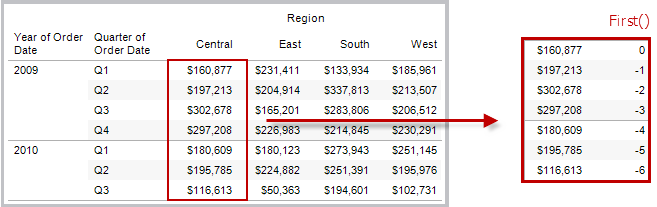#### Example

When the current row index is 3, ```FIRST() = -2```.

### INDEX( )

Returns the index of the current row in the partition, without any sorting with regard to value. The first row index starts at 1. For example, the table below shows quarterly sales. When INDEX() is computed within the Date partition, the index of each row is 1, 2, 3, 4..., etc.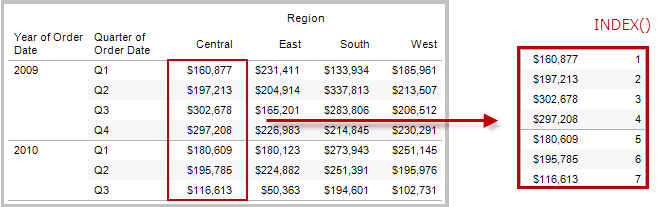#### Example

For the third row in the partition, `INDEX() = 3`.

### LAST( )

Returns the number of rows from the current row to the last row in the partition. For example, the table below shows quarterly sales. When LAST() is computed within the Date partition, the offset of the last row from the second row is 5.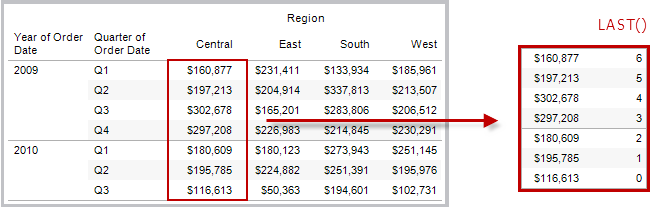#### Example

When the current row index is 3 of 7, `LAST() = 4`.

### LOOKUP(expression, [offset])

Returns the value of the expression in a target row, specified as a relative offset from the current row. Use FIRST() + n and LAST() - n as part of your offset definition for a target relative to the first/last rows in the partition. If `offset` is omitted, the row to compare to can be set on the field menu. This function returns NULL if the target row cannot be determined.

The view below shows quarterly sales. When `LOOKUP (SUM(Sales), 2)` is computed within the Date partition, each row shows the sales value from 2 quarters into the future.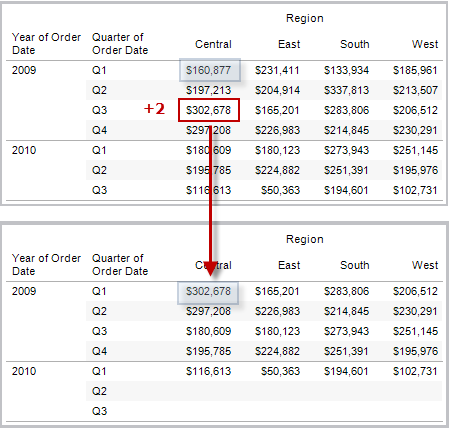#### Example

```LOOKUP(SUM([Profit]), FIRST()+2)``` computes the SUM(Profit) in the third row of the partition.

### MODEL_EXTENSION_BOOL (model_name, arguments, expression)

Returns the boolean result of an expression as calculated by a named model deployed on a TabPy external service.

Model_name is the name of the deployed analytics model you want to use.

Each argument is a single string that sets the input values that the deployed model accepts, and is defined by the analytics model.

Use expressions to define the values that are sent from Tableau to the analytics model. Be sure to use aggregation functions (SUM, AVG, etc.) to aggregate the results.

When using the function, the data types and order of the expressions must match that of the input arguments.

#### Example

`MODEL_EXTENSION_BOOL ("isProfitable","inputSales", "inputCosts", SUM([Sales]), SUM([Costs]))`

### MODEL_EXTENSION_INT (model_name, arguments, expression)

Returns an integer result of an expression as calculated by a named model deployed on a TabPy external service.

Model_name is the name of the deployed analytics model you want to use.

Each argument is a single string that sets the input values that the deployed model accepts, and is defined by the analytics model.

Use expressions to define the values that are sent from Tableau to the analytics model. Be sure to use aggregation functions (SUM, AVG, etc.) to aggregate the results.

When using the function, the data types and order of the expressions must match that of the input arguments.

#### Example

`MODEL_EXTENSION_INT ("getPopulation", "inputCity", "inputState", MAX([City]), MAX ([State]))`

### MODEL_EXTENSION_REAL (model_name, arguments, expression)

Returns a real result of an expression as calculated by a named model deployed on a TabPy external service.

Model_name is the name of the deployed analytics model you want to use.

Each argument is a single string that sets the input values that the deployed model accepts, and is defined by the analytics model.

Use expressions to define the values that are sent from Tableau to the analytics model. Be sure to use aggregation functions (SUM, AVG, etc.) to aggregate the results.

When using the function, the data types and order of the expressions must match that of the input arguments.

#### Example

`MODEL_EXTENSION_REAL ("profitRatio", "inputSales", "inputCosts", SUM([Sales]), SUM([Costs]))`

### MODEL_EXTENSION_STRING (model_name, arguments, expression)

Returns the string result of an expression as calculated by a named model deployed on a TabPy external service.

Model_name is the name of the deployed analytics model you want to use.

Each argument is a single string that sets the input values that the deployed model accepts, and is defined by the analytics model.

Use expressions to define the values that are sent from Tableau to the analytics model. Be sure to use aggregation functions (SUM, AVG, etc.) to aggregate the results.

When using the function, the data types and order of the expressions must match that of the input arguments.

#### Example

`MODEL_EXTENSION_STR ("mostPopulatedCity", "inputCountry", "inputYear", MAX ([Country]), MAX([Year]))`

### MODEL_PERCENTILE(target_expression, predictor_expression(s))

Returns the probability (between 0 and 1) of the expected value being less than or equal to the observed mark, defined by the target expression and other predictors. This is the Posterior Predictive Distribution Function, also known as the Cumulative Distribution Function (CDF).

This function is the inverse of MODEL_QUANTILE. For information on predictive modeling functions, see How Predictive Modeling Functions Work in Tableau.

#### Example

The following formula returns the quantile of the mark for sum of sales, adjusted for count of orders.

`MODEL_PERCENTILE(SUM([Sales]), COUNT([Orders]))`

### MODEL_QUANTILE(quantile, target_expression, predictor_expression(s))

Returns a target numeric value within the probable range defined by the target expression and other predictors, at a specified quantile. This is the Posterior Predictive Quantile.

This function is the inverse of MODEL_PERCENTILE. For information on predictive modeling functions, see How Predictive Modeling Functions Work in Tableau.

#### Example

The following formula returns the median (0.5) predicted sum of sales, adjusted for count of orders.

`MODEL_QUANTILE(0.5, SUM([Sales]), COUNT([Orders]))`

### PREVIOUS_VALUE(expression)

Returns the value of this calculation in the previous row. Returns the given expression if the current row is the first row of the partition.

#### Example

`SUM([Profit]) * PREVIOUS_VALUE(1)` computes the running product of SUM(Profit).

### RANK(expression, ['asc' | 'desc'])

Returns the standard competition rank for the current row in the partition. Identical values are assigned an identical rank. Use the optional `'asc' | 'desc'` argument to specify ascending or descending order. The default is descending.

With this function, the set of values (6, 9, 9, 14) would be ranked (4, 2, 2, 1).

Nulls are ignored in ranking functions. They are not numbered and they do not count against the total number of records in percentile rank calculations.

For information on different ranking options, see Rank calculation.

#### Example

The following image shows the effect of the various ranking functions (RANK, RANK_DENSE, RANK_MODIFIED, RANK_PERCENTILE, and RANK_UNIQUE) on a set of values. The data set contains information on 14 students (StudentA through StudentN); the Age column shows the current age of each student (all students are between 17 and 20 years of age). The remaining columns show the effect of each rank function on the set of age values, always assuming the default order (ascending or descending) for the function.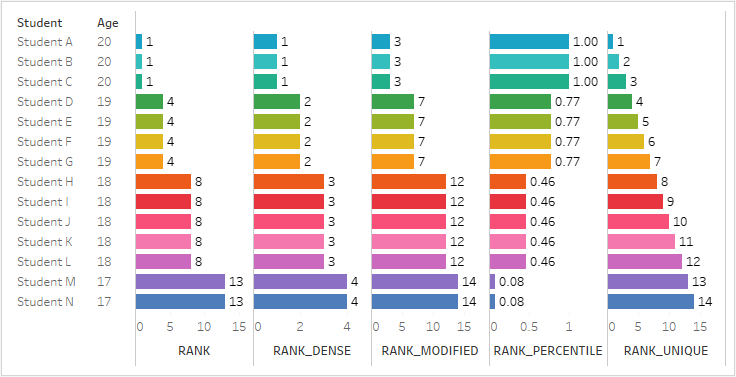### RANK_DENSE(expression, ['asc' | 'desc'])

Returns the dense rank for the current row in the partition. Identical values are assigned an identical rank, but no gaps are inserted into the number sequence. Use the optional `'asc' | 'desc'` argument to specify ascending or descending order. The default is descending.

With this function, the set of values (6, 9, 9, 14) would be ranked (3, 2, 2, 1).

Nulls are ignored in ranking functions. They are not numbered and they do not count against the total number of records in percentile rank calculations.

For information on different ranking options, see Rank calculation.

### RANK_MODIFIED(expression, ['asc' | 'desc'])

Returns the modified competition rank for the current row in the partition. Identical values are assigned an identical rank. Use the optional `'asc' | 'desc'` argument to specify ascending or descending order. The default is descending.

With this function, the set of values (6, 9, 9, 14) would be ranked (4, 3, 3, 1).

Nulls are ignored in ranking functions. They are not numbered and they do not count against the total number of records in percentile rank calculations.

For information on different ranking options, see Rank calculation.

### RANK_PERCENTILE(expression, ['asc' | 'desc'])

Returns the percentile rank for the current row in the partition. Use the optional `'asc' | 'desc'` argument to specify ascending or descending order. The default is ascending.

With this function, the set of values (6, 9, 9, 14) would be ranked (0.00, 0.67, 0.67, 1.00).

Nulls are ignored in ranking functions. They are not numbered and they do not count against the total number of records in percentile rank calculations.

For information on different ranking options, see Rank calculation.

### RANK_UNIQUE(expression, ['asc' | 'desc'])

Returns the unique rank for the current row in the partition. Identical values are assigned different ranks. Use the optional `'asc' | 'desc'` argument to specify ascending or descending order. The default is descending.

With this function, the set of values (6, 9, 9, 14) would be ranked (4, 2, 3, 1).

Nulls are ignored in ranking functions. They are not numbered and they do not count against the total number of records in percentile rank calculations.

For information on different ranking options, see Rank calculation.

### RUNNING_AVG(expression)

Returns the running average of the given expression, from the first row in the partition to the current row.

The view below shows quarterly sales. When `RUNNING_AVG(SUM([Sales])` is computed within the Date partition, the result is a running average of the sales values for each quarter.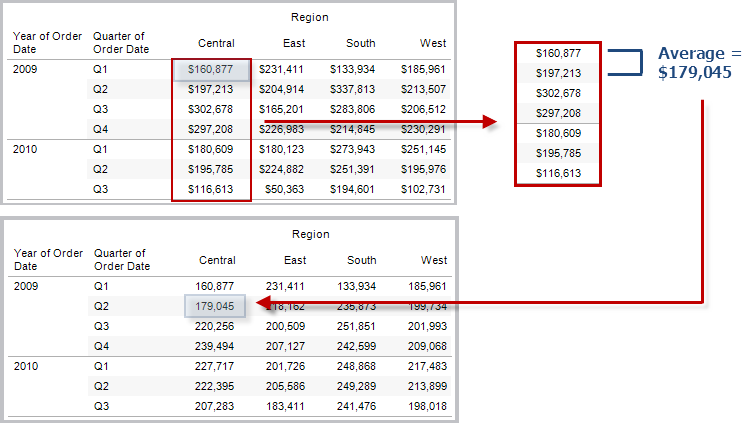#### Example

`RUNNING_AVG(SUM([Profit]))` computes the running average of SUM(Profit).

### RUNNING_COUNT(expression)

Returns the running count of the given expression, from the first row in the partition to the current row.

#### Example

`RUNNING_COUNT(SUM([Profit]))` computes the running count of SUM(Profit).

### RUNNING_MAX(expression)

Returns the running maximum of the given expression, from the first row in the partition to the current row.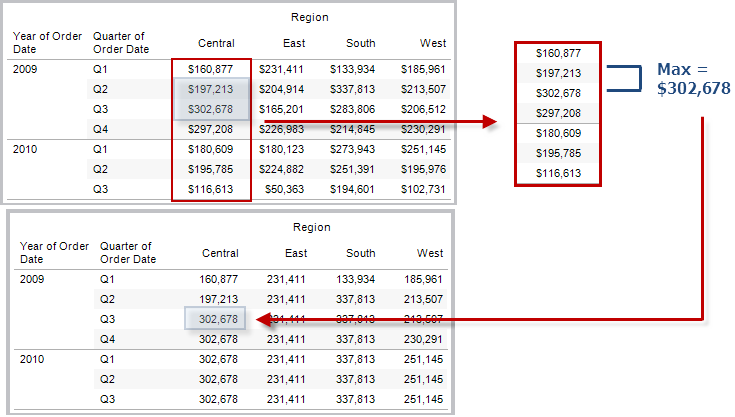#### Example

`RUNNING_MAX(SUM([Profit]))` computes the running maximum of SUM(Profit).

### RUNNING_MIN(expression)

Returns the running minimum of the given expression, from the first row in the partition to the current row.#### Example

`RUNNING_MIN(SUM([Profit]))` computes the running minimum of SUM(Profit).

### RUNNING_SUM(expression)

Returns the running sum of the given expression, from the first row in the partition to the current row.#### Example

`RUNNING_SUM(SUM([Profit]))` computes the running sum of SUM(Profit)

### SIZE()

Returns the number of rows in the partition. For example, the view below shows quarterly sales. Within the Date partition, there are seven rows so the Size() of the Date partition is 7.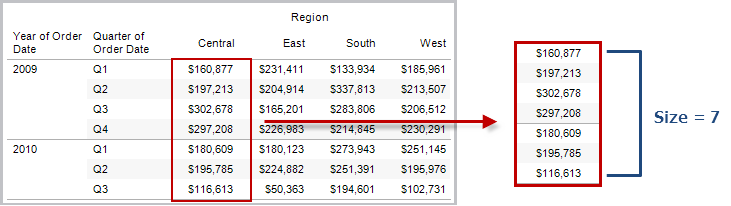#### Example

`SIZE() = 5` when the current partition contains five rows.

### SCRIPT_BOOL

Returns a Boolean result from the specified expression. The expression is passed directly to a running analytics extension service instance.

In R expressions, use .argn (with a leading period) to reference parameters (.arg1, .arg2, etc.).

In Python expressions, use _argn (with a leading underscore).

#### Examples

In this R example, .arg1 is equal to SUM([Profit]):

`SCRIPT_BOOL("is.finite(.arg1)", SUM([Profit]))`

The next example returns True for store IDs in Washington state, and False otherwise. This example could be the definition for a calculated field titled IsStoreInWA.

`SCRIPT_BOOL('grepl(".*_WA", .arg1, perl=TRUE)',ATTR([Store ID]))`

A command for Python would take this form:

`SCRIPT_BOOL("return map(lambda x : x > 0, _arg1)", SUM([Profit]))`

### SCRIPT_INT

Returns an integer result from the specified expression. The expression is passed directly to a running analytics extension service instance.

In R expressions, use .argn (with a leading period) to reference parameters (.arg1, .arg2, etc.)

In Python expressions, use _argn (with a leading underscore).

#### Examples

In this R example, .arg1 is equal to SUM([Profit]):

`SCRIPT_INT("is.finite(.arg1)", SUM([Profit]))`

In the next example, k-means clustering is used to create three clusters:

`SCRIPT_INT('result <- kmeans(data.frame(.arg1,.arg2,.arg3,.arg4), 3);result\$cluster;', SUM([Petal length]), SUM([Petal width]),SUM([Sepal length]),SUM([Sepal width]))`

A command for Python would take this form:

`SCRIPT_INT("return map(lambda x : int(x * 5), _arg1)", SUM([Profit]))`

### SCRIPT_REAL

Returns a real result from the specified expression. The expression is passed directly to a running analytics extension service instance. In

R expressions, use .argn (with a leading period) to reference parameters (.arg1, .arg2, etc.)

In Python expressions, use _argn (with a leading underscore).

#### Examples

In this R example, .arg1 is equal to SUM([Profit]):

`SCRIPT_REAL("is.finite(.arg1)", SUM([Profit]))`

The next example converts temperature values from Celsius to Fahrenheit.

`SCRIPT_REAL('library(udunits2);ud.convert(.arg1, "celsius", "degree_fahrenheit")',AVG([Temperature]))`

A command for Python would take this form:

`SCRIPT_REAL("return map(lambda x : x * 0.5, _arg1)", SUM([Profit]))`

### SCRIPT_STR

Returns a string result from the specified expression. The expression is passed directly to a running analytics extension service instance.

In R expressions, use .argn (with a leading period) to reference parameters (.arg1, .arg2, etc.)

In Python expressions, use _argn (with a leading underscore).

#### Examples

In this R example, .arg1 is equal to SUM([Profit]):

`SCRIPT_STR("is.finite(.arg1)", SUM([Profit]))`

The next example extracts a state abbreviation from a more complicated string (in the original form 13XSL_CA, A13_WA):

`SCRIPT_STR('gsub(".*_", "", .arg1)',ATTR([Store ID]))`

A command for Python would take this form:

`SCRIPT_STR("return map(lambda x : x[:2], _arg1)", ATTR([Region]))`

### TOTAL(expression)

Returns the total for the given expression in a table calculation partition.

#### Example

Assume you are starting with this view: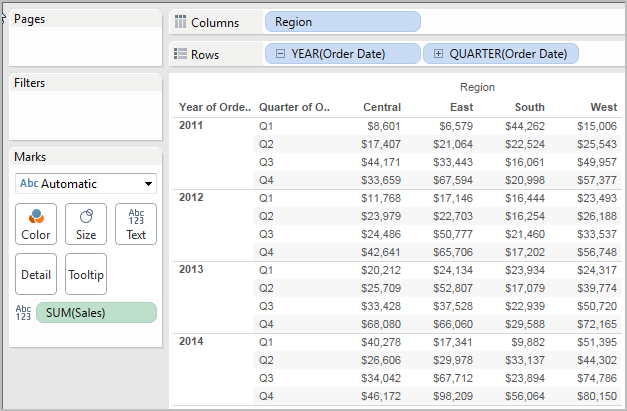You open the calculation editor and create a new field which you name Totality: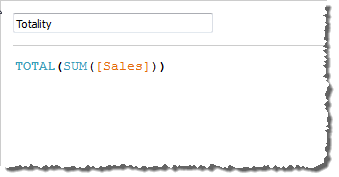You then drop Totality on Text, to replace SUM(Sales). Your view changes such that it sums values based on the default Compute Using value:This raises the question, What is the default Compute Using value? If you right-click (Control-click on a Mac) Totality in the Data pane and choose Edit, there is now an additional bit of information available: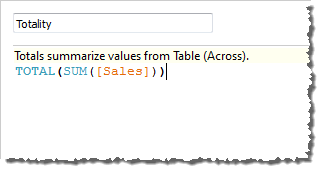The default Compute Using value is Table (Across). The result is that Totality is summing the values across each row of your table. Thus, the value that you see across each row is the sum of the values from the original version of the table.

The values in the 2011/Q1 row in the original table were \$8601, \$6579, \$44262, and \$15006. The values in the table after Totality replaces SUM(Sales) are all \$74,448, which is the sum of the four original values.

Notice the triangle next to Totality after you drop it on Text:This indicates that this field is using a table calculation. You can right-click the field and choose Edit Table Calculation to redirect your function to a different Compute Using value. For example, you could set it to Table (Down). In that case, your table would look like this: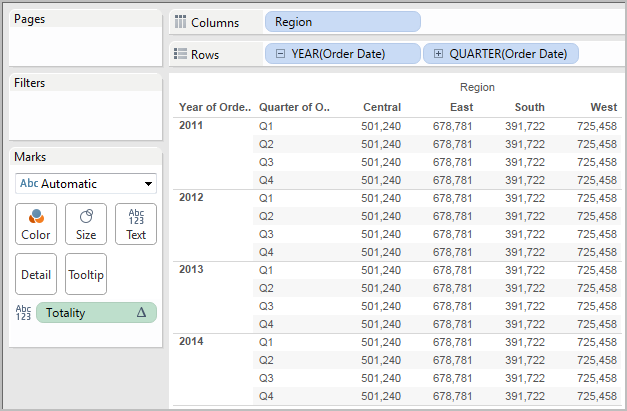### WINDOW_AVG(expression, [start, end])

Returns the average of the expression within the window. The window is defined by means of offsets from the current row. Use FIRST()+n and LAST()-n for offsets from the first or last row in the partition. If the start and end are omitted, the entire partition is used.

For example, the view below shows quarterly sales. A window average within the Date partition returns the average sales across all dates.#### Example

`WINDOW_AVG(SUM([Profit]), FIRST()+1, 0)` computes the average of SUM(Profit) from the second row to the current row.

### WINDOW_CORR(expression1, expression2, [start, end])

Returns the Pearson correlation coefficient of two expressions within the window. The window is defined as offsets from the current row. Use FIRST()+n and LAST()-n for offsets from the first or last row in the partition. If start and end are omitted, the entire partition is used.

The Pearson correlation measures the linear relationship between two variables. Results range from -1 to +1 inclusive, where 1 denotes an exact positive linear relationship, as when a positive change in one variable implies a positive change of corresponding magnitude in the other, 0 denotes no linear relationship between the variance, and −1 is an exact negative relationship.

There is an equivalent aggregation fuction: CORR. See Tableau Functions (Alphabetical)(Link opens in a new window).

#### Example

The following formula returns the Pearson correlation of SUM(Profit) and SUM(Sales) from the five previous rows to the current row.

`WINDOW_CORR(SUM[Profit]), SUM([Sales]), -5, 0)`

### WINDOW_COUNT(expression, [start, end])

Returns the count of the expression within the window. The window is defined by means of offsets from the current row. Use FIRST()+n and LAST()-n for offsets from the first or last row in the partition. If the start and end are omitted, the entire partition is used.

#### Example

`WINDOW_COUNT(SUM([Profit]), FIRST()+1, 0)` computes the count of SUM(Profit) from the second row to the current row

### WINDOW_COVAR(expression1, expression2, [start, end])

Returns the sample covariance of two expressions within the window. The window is defined as offsets from the current row. Use FIRST()+n and LAST()-n for offsets from the first or last row in the partition. If the start and end arguments are omitted, the window is the entire partition.

Sample covariance uses the number of non-null data points n - 1 to normalize the covariance calculation, rather than n, which is used by the population covariance (with the WINDOW_COVARP function). Sample covariance is the appropriate choice when the data is a random sample that is being used to estimate the covariance for a larger population.

There is an equivalent aggregation fuction: COVAR. See Tableau Functions (Alphabetical)(Link opens in a new window).

#### Example

The following formula returns the sample covariance of SUM(Profit) and SUM(Sales) from the two previous rows to the current row.

`WINDOW_COVAR(SUM([Profit]), SUM([Sales]), -2, 0)`

### WINDOW_COVARP(expression1, expression2, [start, end])

Returns the population covariance of two expressions within the window. The window is defined as offsets from the current row. Use FIRST()+n and LAST()-n for offsets from the first or last row in the partition. If start and end are omitted, the entire partition is used.

Population covariance is sample covariance multiplied by (n-1)/n, where n is the total number of non-null data points. Population covariance is the appropriate choice when there is data available for all items of interest as opposed to when there is only a random subset of items, in which case sample covariance (with the WINDOW_COVAR function) is appropriate.

There is an equivalent aggregation fuction: COVARP. Tableau Functions (Alphabetical)(Link opens in a new window).

#### Example

The following formula returns the population covariance of SUM(Profit) and SUM(Sales) from the two previous rows to the current row.

`WINDOW_COVARP(SUM([Profit]), SUM([Sales]), -2, 0)`

### WINDOW_MEDIAN(expression, [start, end])

Returns the median of the expression within the window. The window is defined by means of offsets from the current row. Use FIRST()+n and LAST()-n for offsets from the first or last row in the partition. If the start and end are omitted, the entire partition is used.

For example, the view below shows quarterly profit. A window median within the Date partition returns the median profit across all dates.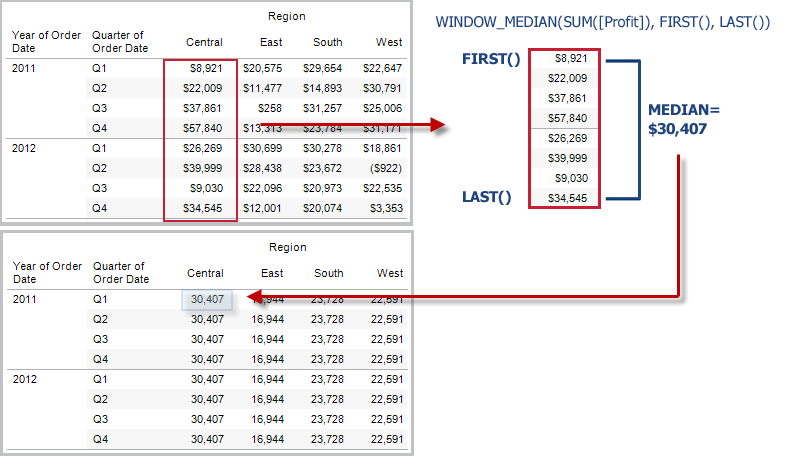#### Example

`WINDOW_MEDIAN(SUM([Profit]), FIRST()+1, 0)` computes the median of SUM(Profit) from the second row to the current row.

### WINDOW_MAX(expression, [start, end])

Returns the maximum of the expression within the window. The window is defined by means of offsets from the current row. Use FIRST()+n and LAST()-n for offsets from the first or last row in the partition. If the start and end are omitted, the entire partition is used.

For example, the view below shows quarterly sales. A window maximum within the Date partition returns the maximum sales across all dates.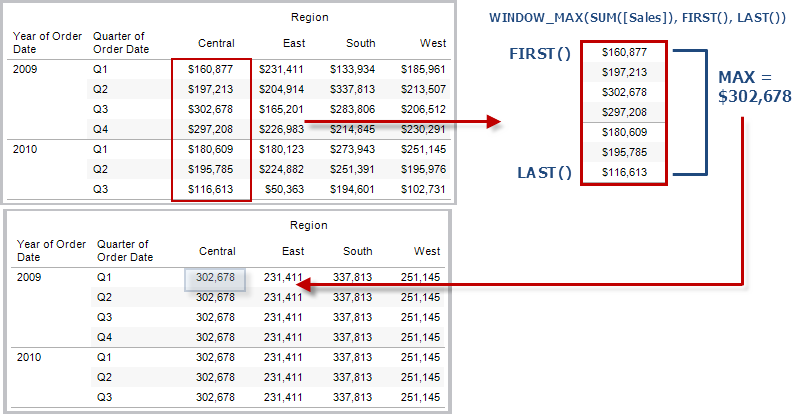#### Example

`WINDOW_MAX(SUM([Profit]), FIRST()+1, 0)` computes the maximum of SUM(Profit) from the second row to the current row.

### WINDOW_MIN(expression, [start, end])

Returns the minimum of the expression within the window. The window is defined by means of offsets from the current row. Use FIRST()+n and LAST()-n for offsets from the first or last row in the partition. If the start and end are omitted, the entire partition is used.

For example, the view below shows quarterly sales. A window minimum within the Date partition returns the minimum sales across all dates.#### Example

`WINDOW_MIN(SUM([Profit]), FIRST()+1, 0)` computes the minimum of SUM(Profit) from the second row to the current row.

### WINDOW_PERCENTILE(expression, number, [start, end])

Returns the value corresponding to the specified percentile within the window. The window is defined by means of offsets from the current row. Use FIRST()+n and LAST()-n for offsets from the first or last row in the partition. If the start and end are omitted, the entire partition is used.

#### Example

`WINDOW_PERCENTILE(SUM([Profit]), 0.75, -2, 0)` returns the 75th percentile for SUM(Profit) from the two previous rows to the current row.

### WINDOW_STDEV(expression, [start, end])

Returns the sample standard deviation of the expression within the window. The window is defined by means of offsets from the current row. Use FIRST()+n and LAST()-n for offsets from the first or last row in the partition. If the start and end are omitted, the entire partition is used.

#### Example

`WINDOW_STDEV(SUM([Profit]), FIRST()+1, 0)` computes the standard deviation of SUM(Profit) from the second row to the current row.

### WINDOW_STDEVP(expression, [start, end])

Returns the biased standard deviation of the expression within the window. The window is defined by means of offsets from the current row. Use FIRST()+n and LAST()-n for offsets from the first or last row in the partition. If the start and end are omitted, the entire partition is used.

#### Example

`WINDOW_STDEVP(SUM([Profit]), FIRST()+1, 0)` computes the standard deviation of SUM(Profit) from the second row to the current row.

### WINDOW_SUM(expression, [start, end])

Returns the sum of the expression within the window. The window is defined by means of offsets from the current row. Use FIRST()+n and LAST()-n for offsets from the first or last row in the partition. If the start and end are omitted, the entire partition is used.

For example, the view below shows quarterly sales. A window sum computed within the Date partition returns the summation of sales across all quarters.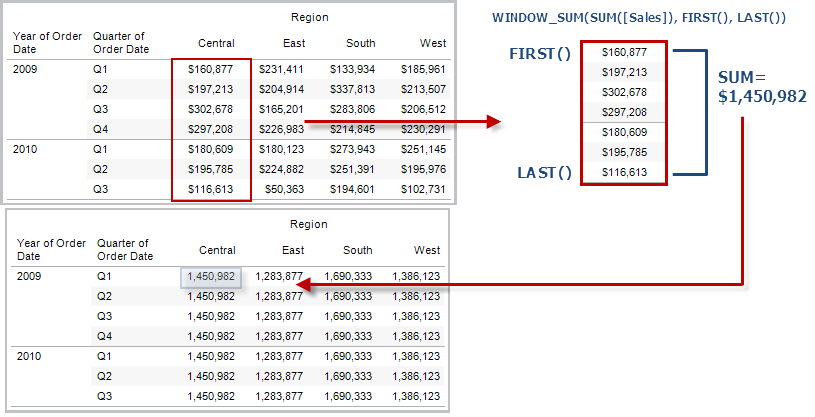#### Example

`WINDOW_SUM(SUM([Profit]), FIRST()+1, 0)` computes the sum of SUM(Profit) from the second row to the current row.

### WINDOW_VAR(expression, [start, end])

Returns the sample variance of the expression within the window. The window is defined by means of offsets from the current row. Use FIRST()+n and LAST()-n for offsets from the first or last row in the partition. If the start and end are omitted, the entire partition is used.

#### Example

`WINDOW_VAR((SUM([Profit])), FIRST()+1, 0)` computes the variance of SUM(Profit) from the second row to the current row.

### WINDOW_VARP(expression, [start, end])

Returns the biased variance of the expression within the window. The window is defined by means of offsets from the current row. Use FIRST()+n and LAST()-n for offsets from the first or last row in the partition. If the start and end are omitted, the entire partition is used.

#### Example

`WINDOW_VARP(SUM([Profit]), FIRST()+1, 0)` computes the variance of SUM(Profit) from the second row to the current row.

Pass-Through functions (RAWSQL)

These RAWSQL pass-through functions can be used to send SQL expressions directly to the database, without first being interpreted by Tableau. If you have custom database functions that Tableau doesn’t know about, you can use these pass-through functions to call these custom functions.

Your database usually will not understand the field names that are shown in Tableau. Because Tableau does not interpret the SQL expressions you include in the pass-through functions, using the Tableau field names in your expression may cause errors. You can use a substitution syntax to insert the correct field name or expression for a Tableau calculation into pass-through SQL. For example, if you had a function that computed the median of a set of values, you could call that function on the Tableau column [Sales] like this:

RAWSQLAGG_REAL(“MEDIAN(%1)”, [Sales])

Because Tableau does not interpret the expression, you must define the aggregation. You can use the RAWSQLAGG functions described below when you are using aggregated expressions.

RAWSQL pass-through functions may not work with extracts or published data sources if they contain relationships.

## RAWSQL Functions

The following RAWSQL functions are available in Tableau.

### RAWSQL_BOOL(“sql_expr”, [arg1], …[argN])

Returns a Boolean result from a given SQL expression. The SQL expression is passed directly to the underlying database. Use %n in the SQL expression as a substitution syntax for database values.

#### Example

In the example, %1 is equal to [Sales] and %2 is equal to [Profit].

RAWSQL_BOOL(“IIF( %1 > %2, True, False)”, [Sales], [Profit])

### RAWSQL_DATE(“sql_expr”, [arg1], …[argN])

Returns a Date result from a given SQL expression. The SQL expression is passed directly to the underlying database. Use %n in the SQL expression as a substitution syntax for database values.

#### Example

In this example, %1 is equal to [Order Date].

```RAWSQL_DATE(“%1”, [Order Date])```

### RAWSQL_DATETIME(“sql_expr”, [arg1], …[argN])

Returns a Date and Time result from a given SQL expression. The SQL expression is passed directly to the underlying database. Use %n in the SQL expression as a substitution syntax for database values. In this example, %1 is equal to [Delivery Date].

#### Example

RAWSQL_DATETIME(“MIN(%1)”, [Delivery Date])

### RAWSQL_INT(“sql_expr”, [arg1], …[argN])

Returns an integer result from a given SQL expression. The SQL expression is passed directly to the underlying database. Use %n in the SQL expression as a substitution syntax for database values. In this example, %1 is equal to [Sales].

#### Example

```RAWSQL_INT(“500 + %1”, [Sales])```

### RAWSQL_REAL(“sql_expr”, [arg1], …[argN])

Returns a numeric result from a given SQL expression that is passed directly to the underlying database. Use %n in the SQL expression as a substitution syntax for database values. In this example, %1 is equal to [Sales]

#### Example

`RAWSQL_REAL(“-123.98 * %1”, [Sales])`

### RAWSQL_SPATIAL

Returns a Spatial from a given SQL expression that is passed directly to the underlying data source. Use %n in the SQL expression as a substitution syntax for database values.

#### Example

In this example, %1 is equal to [Geometry].

`RAWSQL_SPATIAL("%1", [Geometry])`

### RAWSQL_STR(“sql_expr”, [arg1], …[argN])

Returns a string from a given SQL expression that is passed directly to the underlying database. Use %n in the SQL expression as a substitution syntax for database values. In this example, %1 is equal to [Customer Name].

#### Example

`RAWSQL_STR(“%1”, [Customer Name])`

### RAWSQLAGG_BOOL(“sql_expr”, [arg1], …[argN])

Returns a Boolean result from a given aggregate SQL expression. The SQL expression is passed directly to the underlying database. Use %n in the SQL expression as a substitution syntax for database values.

#### Example

In the example, %1 is equal to [Sales] and %2 is equal to [Profit].

RAWSQLAGG_BOOL(“SUM( %1) >SUM( %2)”, [Sales], [Profit])

### RAWSQLAGG_DATE(“sql_expr”, [arg1], …[argN])

Returns a Date result from a given aggregate SQL expression. The SQL expression is passed directly to the underlying database. Use %n in the SQL expression as a substitution syntax for database values. In this example, %1 is equal to [Order Date].

#### Example

```RAWSQLAGG_DATE(“MAX(%1)”, [Order Date])```

### RAWSQLAGG_DATETIME(“sql_expr”, [arg1], …[argN])

Returns a Date and Time result from a given aggregate SQL expression. The SQL expression is passed directly to the underlying database. Use %n in the SQL expression as a substitution syntax for database values. In this example, %1 is equal to [Delivery Date].

#### Example

`RAWSQLAGG_DATETIME(“MIN(%1)”, [Delivery Date])`

### RAWSQLAGG_INT(“sql_expr”, [arg1,] …[argN])

Returns an integer result from a given aggregate SQL expression. The SQL expression is passed directly to the underlying database. Use %n in the SQL expression as a substitution syntax for database values. In this example, %1 is equal to [Sales].

#### Example

```RAWSQLAGG_INT(“500 + SUM(%1)”, [Sales])```

### RAWSQLAGG_REAL(“sql_expr”, [arg1,] …[argN])

Returns a numeric result from a given aggregate SQL expression that is passed directly to the underlying database. Use %n in the SQL expression as a substitution syntax for database values. In this example, %1 is equal to [Sales]

#### Example

`RAWSQLAGG_REAL(“SUM( %1)”, [Sales])`

### RAWSQLAGG_STR(“sql_expr”, [arg1,] …[argN])

Returns a string from a given aggregate SQL expression that is passed directly to the underlying database. Use %n in the SQL expression as a substitution syntax for database values. In this example, %1 is equal to [Discount].

#### Example

```RAWSQLAGG_STR(“AVG(%1)”, [Discount])```

Spatial functions

Spatial functions allow you to perform advanced spatial analysis and combine spatial files with data in other formats like text files or spreadsheets.

#### Description

AREA AREA(Spatial Polygon, "units")

Returns the total surface area of a spatial polygon.

Supported unit names: meters ("meters," "metres" "m"), kilometers ("kilometers," "kilometres," "km"), miles ("miles" or "mi"), feet ("feet," "ft").

BUFFER `BUFFER(Spatial Point, distance, "units")`

Returns a polygon shape with a radius determined by the distance and unit values defined in the calculation.

Note: The Buffer calculation will only work with a Point spatial object.

BUFFER supports the same unit names as the DISTANCE function.

DISTANCE

`DISTANCE(<Spatial Point1>,<Spatial Point2>,"<units>")`

Returns distance measurement between two points in a specified unit. Supported unit names: meters ("meters," "metres" "m"), kilometers ("kilometers," "kilometres," "km"), miles ("miles" or "mi"), feet ("feet," "ft").

This function can only be created with a live connection and will continue to work when a data source is converted to an extract.

Example:

`DISTANCE([Origin MakePoint],[Destination MakePoint], "km")`

INTERSECTS INTERSECTS (<geometry1>,<geometry2>)

Returns a Boolean (True/False) indicating if two geometries overlap in space.

Supported combinations: point/polygon, line/polygon, and polygon/polygon.

MAKELINE `MAKELINE(<Spatial Point1>,<Spatial Point2>)`

Generates a line mark between two points; useful for building origin-destination maps.

Examples:

`MAKELINE(OriginPoint, DestinationPoint)`

`MAKELINE(MAKEPOINT(OriginLat],[OriginLong]),MAKEPOINT([DestinationLat],[DestinationLong])`

MAKEPOINT `MAKEPOINT(<latitude>, <longitude>) `

Converts data from latitude and longitude columns into spatial objects.

You can use MAKEPOINT to spatially-enable a data source so that it can be joined with a spatial file using a spatial join. For more information, see Join Spatial Files in Tableau .

To use MAKEPOINT, your data must contain latitude and longitude coordinates.

Example:

`MAKEPOINT([AirportLatitude],[AirportLongitude])`

MAKEPOINT(X,Y, SRID)

`MAKEPOINT(<coordinatesX>, <coordinatesY>, <SRID>`

Converts data from projected geographic coordinates into spatial objects. SRID is a spatial reference identifier that uses ESPG reference system codes to specify coordinate systems. If SRID is not specified, WGS84 is assumed and parameters are treated as latitude/longitude in degrees.

This function can only be created with a live connection and will continue to work when a data source is converted to an extract.

Example:

`MAKEPOINT([Xcoord],[Ycoord],3493)`

LENGTH `Length (Spatial lines)` Calculates the combined length of all line strings in the spatial parameter.
OUTLINE `Outline (Spatial geometry)` Turns polygons (including those within multipolygons) into line strings. For example, you could separate outline from fill if you put the outline on another layer.
SHAPETYPE `ShapeType (Spatial geometry)` Returns a string describing the structure of the spatial geometry, such as `Empty`, `Point`, `MultiPoint`, `LineString`, `MultiLinestring`, `Polygon`, `MultiPolygon`, `Mixed`, and `unsupported`.

#### Regular Expressions

##### REGEXP_REPLACE(string, pattern, replacement)

Returns a copy of the given string where the regular expression pattern is replaced by the replacement string. This function is available for Text File, Hadoop Hive, Google BigQuery, PostgreSQL, Tableau Data Extract, Microsoft Excel, Salesforce, Vertica, Pivotal Greenplum, Teradata (version 14.1 and above), Snowflake, and Oracle data sources.

For Tableau data extracts, the pattern and the replacement must be constants.

For information on regular expression syntax, see your data source's documentation. For Tableau extracts, regular expression syntax conforms to the standards of the ICU (International Components for Unicode), an open source project of mature C/C++ and Java libraries for Unicode support, software internationalization, and software globalization. See the Regular Expressions(Link opens in a new window) page in the online ICU User Guide.

##### Example

REGEXP_REPLACE('abc 123', '\s', '-') = 'abc-123'

##### REGEXP_MATCH(string, pattern)

Returns true if a substring of the specified string matches the regular expression pattern. This function is available for Text File, Google BigQuery, PostgreSQL, Tableau Data Extract, Microsoft Excel, Salesforce, Vertica, Pivotal Greenplum, Teradata (version 14.1 and above), Impala 2.3.0 (through Cloudera Hadoop data sources), Snowflake, and Oracle data sources.

For Tableau data extracts, the pattern must be a constant.

For information on regular expression syntax, see your data source's documentation. For Tableau extracts, regular expression syntax conforms to the standards of the ICU (International Components for Unicode), an open source project of mature C/C++ and Java libraries for Unicode support, software internationalization, and software globalization. See the Regular Expressions(Link opens in a new window) page in the online ICU User Guide.

##### Example

REGEXP_MATCH('-(.[The.Market])-','\[\s*(\w*\.)(\w*\s*\])')=true

#### REGEXP_EXTRACT(string, pattern)

Returns the portion of the string that matches the regular expression pattern. This function is available for Text File, Hadoop Hive, Google BigQuery, PostgreSQL, Tableau Data Extract, Microsoft Excel, Salesforce, Vertica, Pivotal Greenplum, Teradata (version 14.1 and above), Snowflake, and Oracle data sources.

For Tableau data extracts, the pattern must be a constant.

For information on regular expression syntax, see your data source's documentation. For Tableau extracts, regular expression syntax conforms to the standards of the ICU (International Components for Unicode), an open source project of mature C/C++ and Java libraries for Unicode support, software internationalization, and software globalization. See the Regular Expressions(Link opens in a new window) page in the online ICU User Guide.

##### Example

REGEXP_EXTRACT('abc 123', '[a-z]+\s+(\d+)') = '123'

##### REGEXP_EXTRACT_NTH(string, pattern, index)

Returns the portion of the string that matches the regular expression pattern. The substring is matched to the nth capturing group, where n is the given index. If index is 0, the entire string is returned. This function is available for Text File, PostgreSQL, Tableau Data Extract, Microsoft Excel, Salesforce, Vertica, Pivotal Greenplum, Teradata (version 14.1 and above), and Oracle data sources.

For Tableau data extracts, the pattern must be a constant.

For information on regular expression syntax, see your data source's documentation. For Tableau extracts, regular expression syntax conforms to the standards of the ICU (International Components for Unicode), an open source project of mature C/C++ and Java libraries for Unicode support, software internationalization, and software globalization. See the Regular Expressions(Link opens in a new window) page in the online ICU User Guide.

#### Example

REGEXP_EXTRACT_NTH('abc 123', '([a-z]+)\s+(\d+)', 2) = '123'

Note: Only the PARSE_URL and PARSE_URL_QUERY functions are available for Cloudera Impala data sources.

##### GET_JSON_OBJECT(JSON string, JSON path)

Returns the JSON object within the JSON string based on the JSON path.

##### PARSE_URL(string, url_part)

Returns a component of the given URL string where the component is defined by url_part. Valid url_part values include: 'HOST', 'PATH', 'QUERY', 'REF', 'PROTOCOL', 'AUTHORITY', 'FILE' and 'USERINFO'.

##### Example

PARSE_URL('http://www.tableau.com', 'HOST') = 'www.tableau.com'

##### PARSE_URL_QUERY(string, key)

Returns the value of the specified query parameter in the given URL string. The query parameter is defined by the key.

##### Example

PARSE_URL_QUERY('http://www.tableau.com?page=1&cat=4', 'page') = '1'

##### XPATH_BOOLEAN(XML string, XPath expression string)

Returns true if the XPath expression matches a node or evaluates to true.

##### Example

XPATH_BOOLEAN('<values> <value id="0">1</value><value id="1">5</value>', 'values/value[@id="1"] = 5') = true

##### XPATH_DOUBLE(XML string, XPath expression string)

Returns the floating-point value of the XPath expression.

##### Example

XPATH_DOUBLE('<values><value>1.0</value><value>5.5</value> </values>', 'sum(value/*)') = 6.5

##### XPATH_FLOAT(XML string, XPath expression string)

Returns the floating-point value of the XPath expression.

##### Example

XPATH_FLOAT('<values><value>1.0</value><value>5.5</value> </values>','sum(value/*)') = 6.5

##### XPATH_INT(XML string, XPath expression string)

Returns the numerical value of the XPath expression, or zero if the XPath expression cannot evaluate to a number.

##### Example

XPATH_INT('<values><value>1</value><value>5</value> </values>','sum(value/*)') = 6

##### XPATH_LONG(XML string, XPath expression string)

Returns the numerical value of the XPath expression, or zero if the XPath expression cannot evaluate to a number.

##### Example

XPATH_LONG('<values><value>1</value><value>5</value> </values>','sum(value/*)') = 6

##### XPATH_SHORT(XML string, XPath expression string)

Returns the numerical value of the XPath expression, or zero if the XPath expression cannot evaluate to a number.

##### Example

XPATH_SHORT('<values><value>1</value><value>5</value> </values>','sum(value/*)') = 6

##### XPATH_STRING(XML string, XPath expression string)

Returns the text of the first matching node.

##### Example

XPATH_STRING('<sites ><url domain="org">http://www.w3.org</url> <url domain="com">http://www.tableau.com</url></sites>', 'sites/url[@domain="com"]') = 'http://www.tableau.com'

##### DOMAIN(string_url)

Given a URL string, returns the domain as a string.

##### GROUP_CONCAT(expression)

Concatenates values from each record into a single comma-delimited string. This function acts like a SUM() for strings.

##### Example

GROUP_CONCAT(Region) = "Central,East,West"

##### HOST(string_url)

Given a URL string, returns the host name as a string.

##### LOG2(number)

Returns the logarithm base 2 of a number.

##### Example

LOG2(16) = '4.00'

##### LTRIM_THIS(string, string)

Returns the first string with any leading occurrence of the second string removed.

##### Example

LTRIM_THIS('[-Sales-]','[-') = 'Sales-]'

##### RTRIM_THIS(string, string)

Returns the first string with any trailing occurrence of the second string removed.

##### Example

RTRIM_THIS('[-Market-]','-]') = '[-Market'

##### TIMESTAMP_TO_USEC(expression)

Converts a TIMESTAMP data type to a UNIX timestamp in microseconds.

##### Example

TIMESTAMP_TO_USEC(#2012-10-01 01:02:03#)=1349053323000000

##### USEC_TO_TIMESTAMP(expression)

Converts a UNIX timestamp in microsseconds to a TIMESTAMP data type.

##### Example

USEC_TO_TIMESTAMP(1349053323000000) = #2012-10-01 01:02:03#

##### TLD(string_url)

Given a URL string, returns the top level domain plus any country/region domain in the URL.